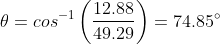Trigonometry : Finding Angles

Example Questions

Example Question #1 : Finding Angles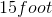plank has one end on the ground and one end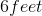off the ground. What is the measure of the angle formed by the plank and the ground?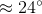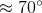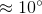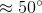Explanation:

The length of the plank becomes the hypotenuse of the triangle, while the distance between the plank and the ground becomes the length of one side. To solve for the angle between the plank and the ground, you must find the value of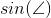. The sine of the angle is the value of the opposite side over the hypotenuse, which are values that we know.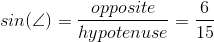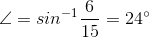Example Question #2 : Finding Angles

Two angles in a triangle are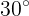and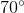. What is the measure of the 3rd angle?There is not enough information to determine the angle measure.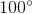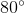Explanation:

The sum of the angles of a triangle is 180˚.

Thus, since the sum of our two angles is 100˚, our missing angle must be,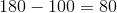Example Question #3 : Finding Angles

If the hypotenuse of a right triangle has a length of 6, and the length of a leg is 2, what is the angle between the hypotenuse and the leg?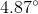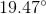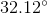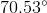Explanation:

The leg must be an adjacent side to the hypotenuse.

Therefore, we can use inverse cosine to solve for the angle.

First write the equation for sine of an angle.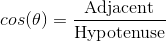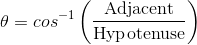Substitute the lengths given and solve for the angle.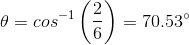Example Question #1 : Finding Angles

A skateboard ramp made so that the rider can gain sufficient speed before a jump is 15 feet high and the ramp is 17 feet long. What is the measure of the angle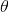between the ramp and the ground?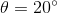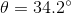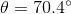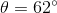Explanation:

For the angle in question we have the opposite side and the hypotenuse given to us. We can use the sine function.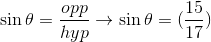Use the inverse sin to find the measure of an angle between these sides: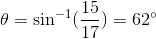Example Question #5 : Finding Angles

What angle does the ramp make with the bottom of the stair?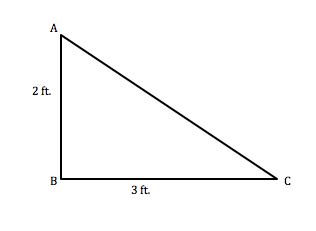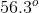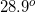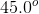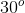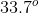Explanation: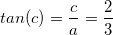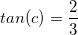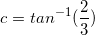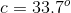Example Question #1 : Solving Triangles

Given that angle A is 23.0o, side a is 1.43 in., and side b is 3.62 in., what is the angle of B?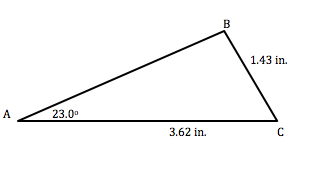70o

90o

55o

81.5o

83.6o

81.5o

Explanation: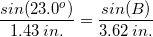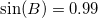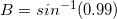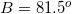Example Question #7 : Finding Angles

Artemis wants to build a ramp to make the entrance to their home more accessible. The angle between the ramp and the ground cannot be more than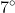steep. Artemis has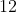feet of space in their yard that the ramp can take up, and the distance between the ground and the house entrance is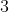feet high. Will Artemis be able to build a ramp that complies with the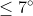standard?

No

Yes

No

Explanation:

Begin the problem by visualizing a diagram of the situation: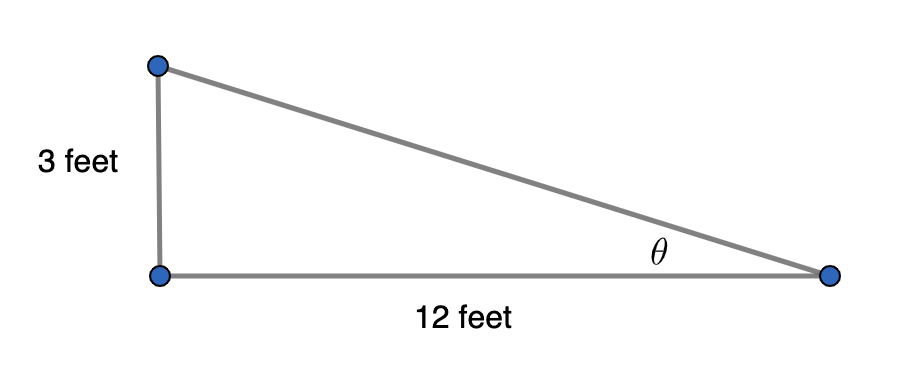We can use inverse trig to solve for the unknown angle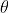.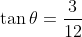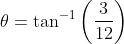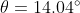Because this angle is larger than, this ramp would not comply with standards.

Example Question #8 : Finding Angles

If the hypotenuse of a right triangle has a length of 42.29 meters, and the length of a leg is 12.88 meters, what is the angle between the hypotenuse and the leg?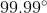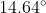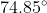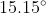Explanation:

The leg must be an adjacent side to the hypotenuse.

Therefore, we can use inverse cosine to solve for the angle.

First write the equation for sine of an angle.Substitute the lengths given and solve for the angle.